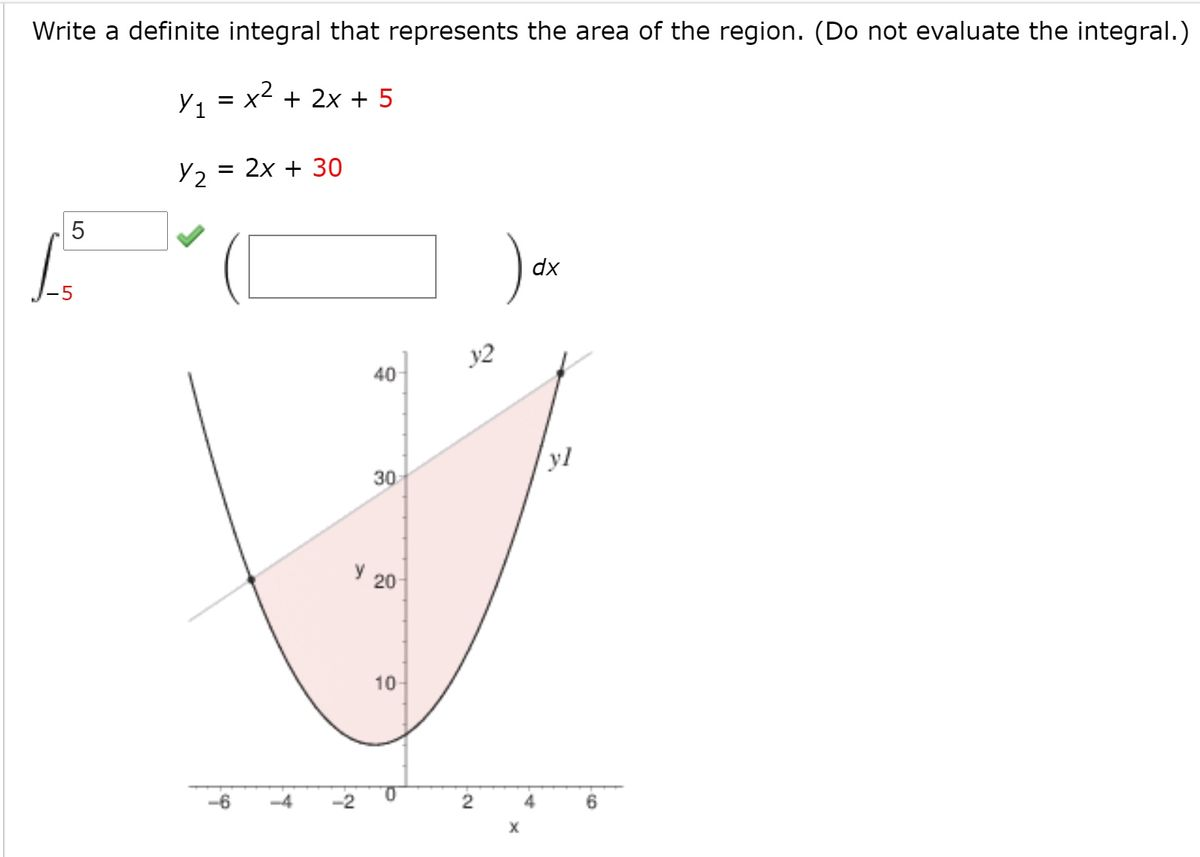# Write a definite integral that represents the area of the region. (Do not evaluate the integral.) y, = x2 + 2x + 5 Y2 = 2x + 30 5 dx y2 40 yl 30 Y 20 10 -6 -2 4

Question
Write a definite integral that represents the area of the region. (Do not evaluate the integral.)
y1 = x2 + 2x + 5
y2 = 2x + 30help_outlineImage TranscriptioncloseWrite a definite integral that represents the area of the region. (Do not evaluate the integral.) y, = x2 + 2x + 5 Y2 = 2x + 30 5 dx y2 40 yl 30 Y 20 10 -6 -2 4 fullscreen

### Want to see this answer and more?

Experts are waiting 24/7 to provide step-by-step solutions in as fast as 30 minutes!*

*Response times may vary by subject and question complexity. Median response time is 34 minutes for paid subscribers and may be longer for promotional offers.
Tagged in
Math
Calculus

### Applications of Integration